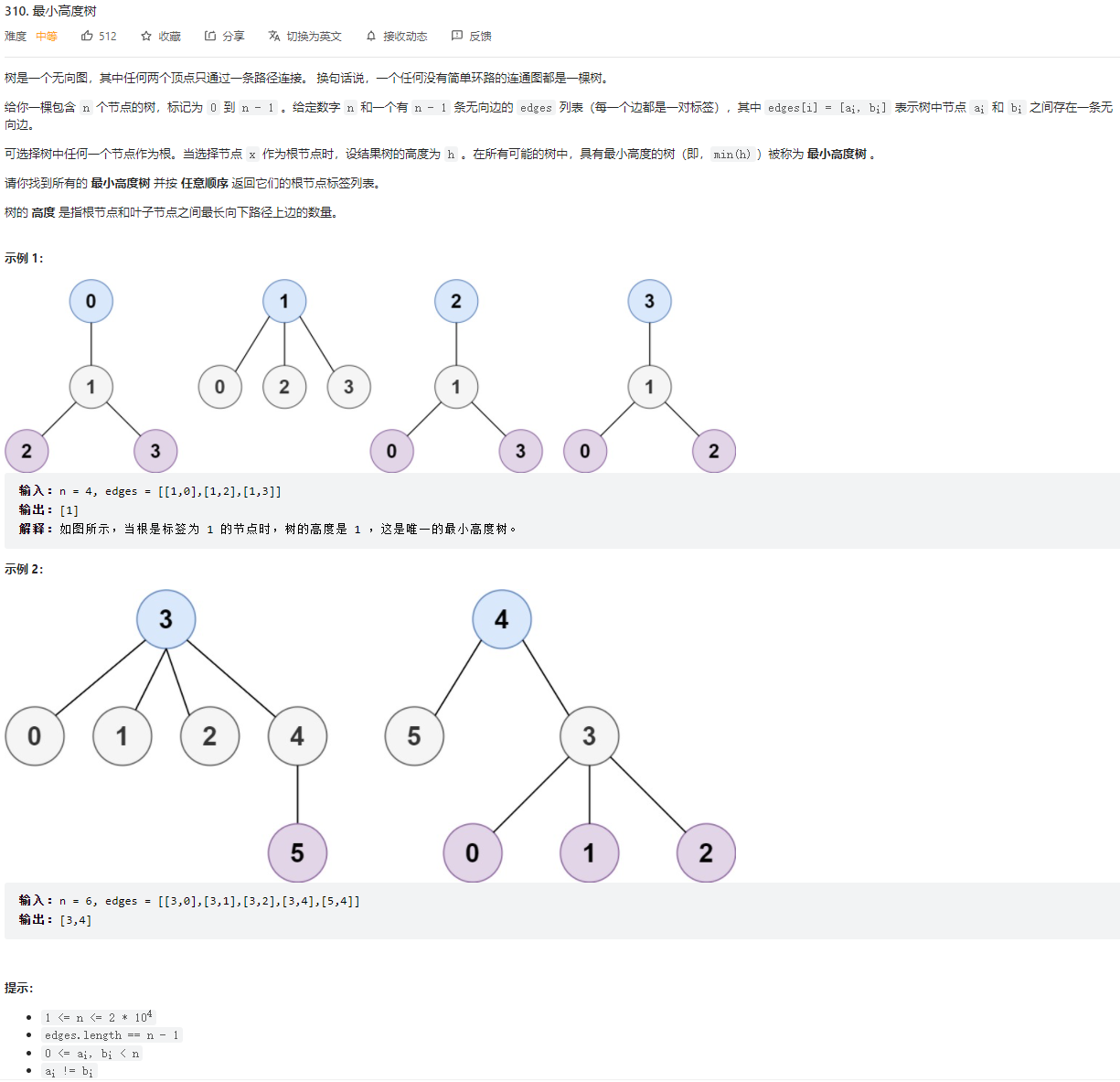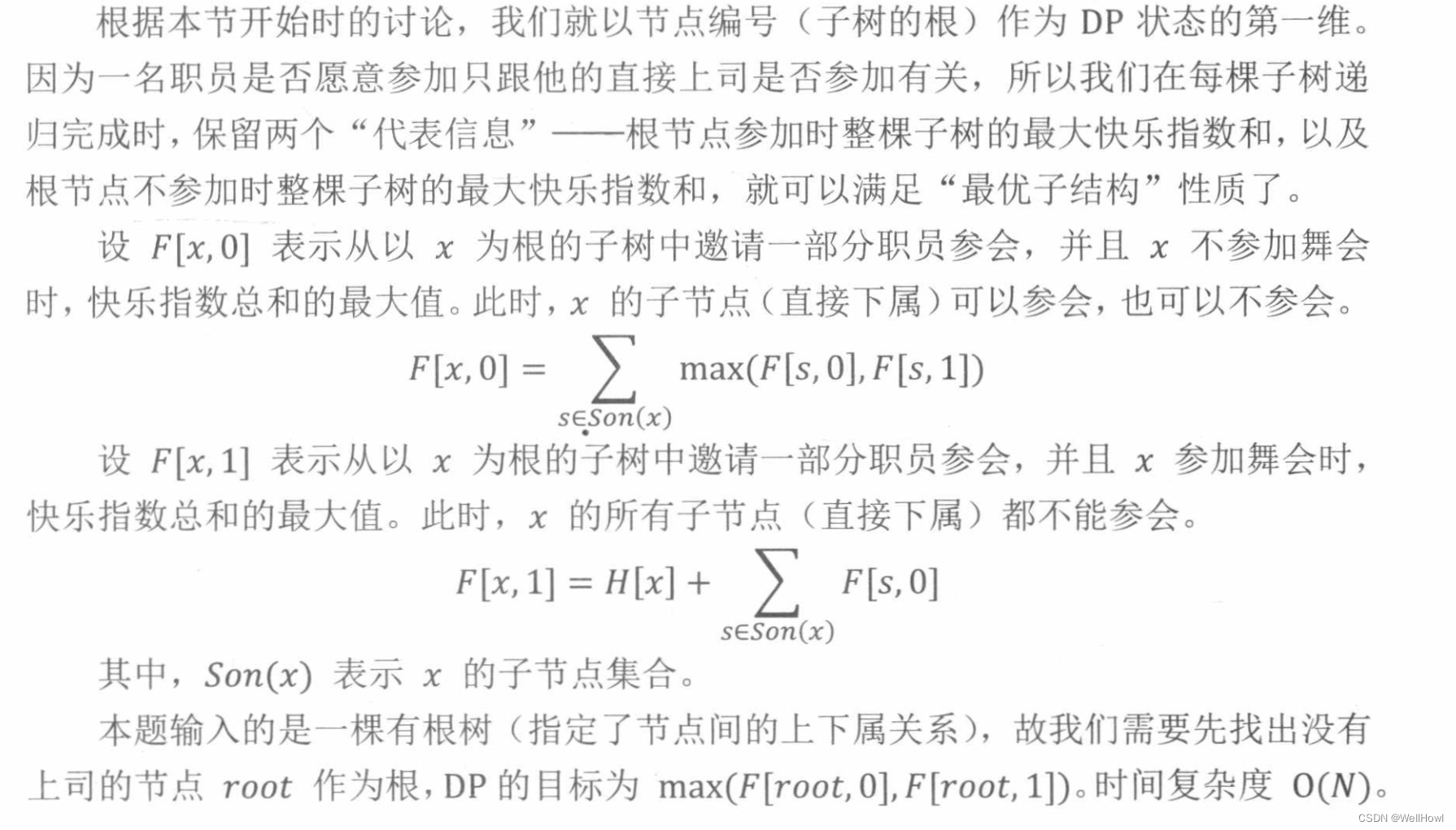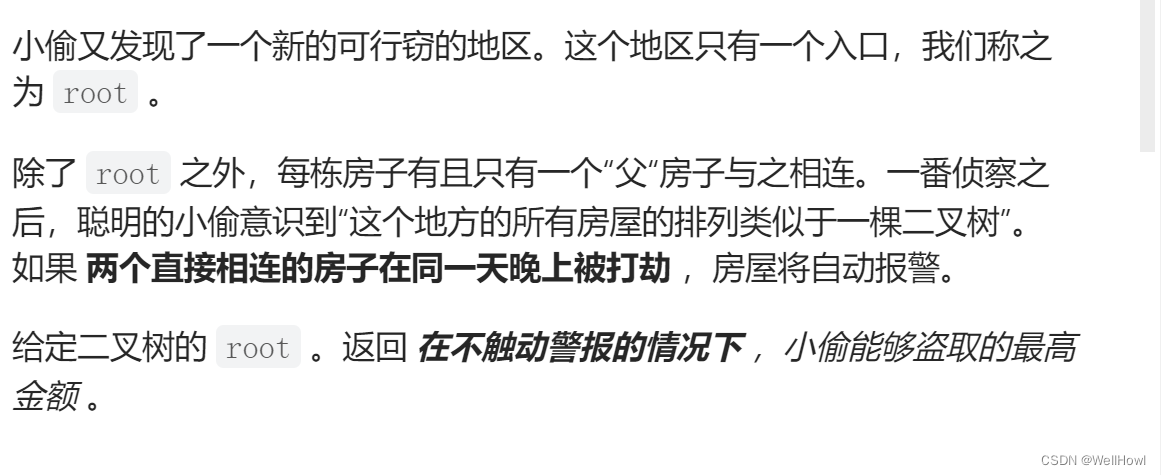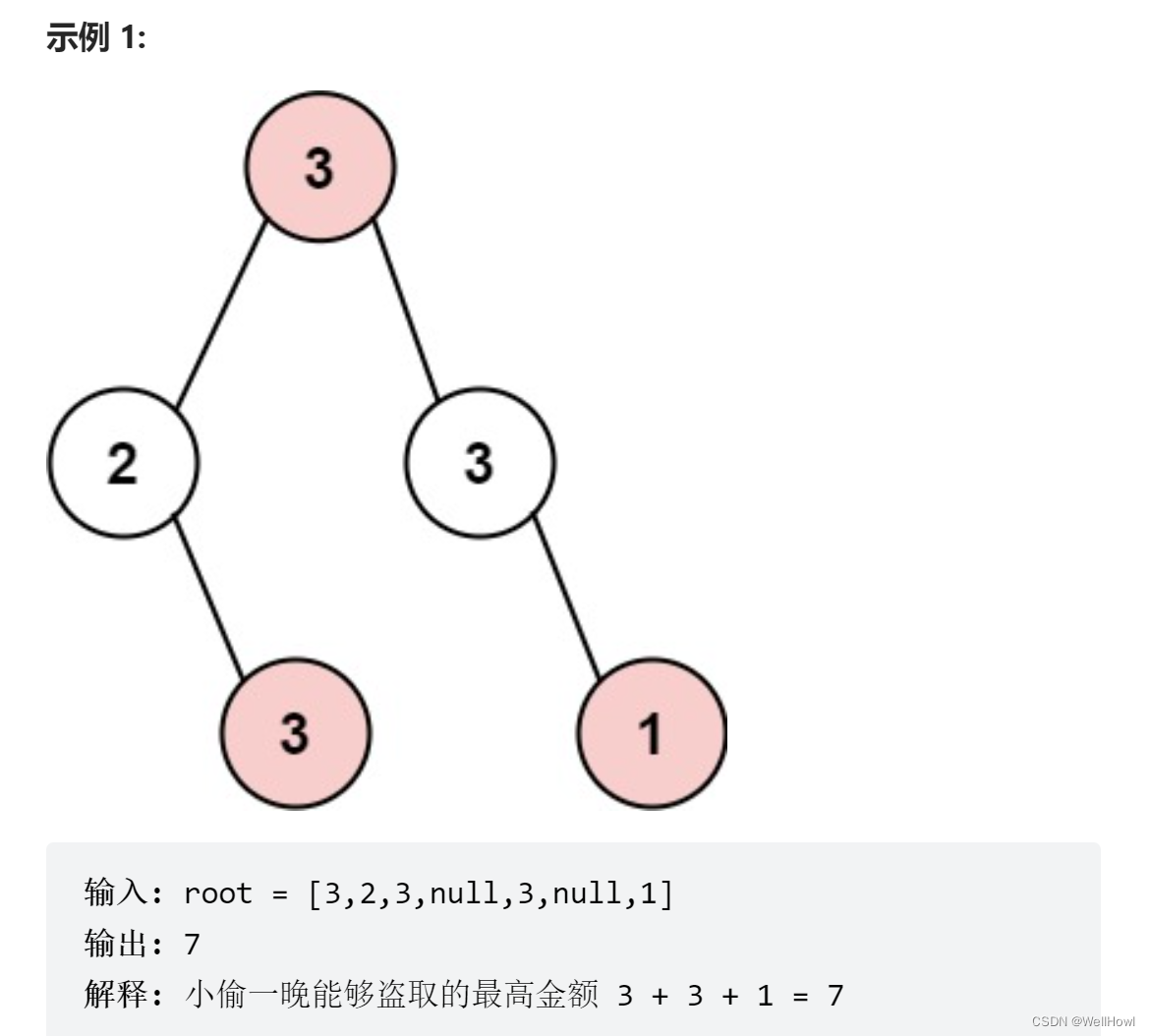• leetcode 树形dp
2021-04-24 21:15:31

L337. 打家劫舍 III利用后序遍历，同时利用无后效性
L124. 二叉树中的最大路径和首先最大路径不一定从根节点开始，有可能根节点恰好位于其中某一环节，仍然利用后序遍历，最终确定，在以根节点为中间环节情况下的最值
L543 二叉树的直径与上面是相同的思路，都是借助了后序遍历，该辅助函数并不直接计算所需要的结果，都是在遍历过程中一一确定；这里也是借助求二叉树的深度进一步求得的。
L687. 最长同值路径
L1372二叉树中的最长交错路径这里变成交叉计数，不完全是后续遍历的形式了
L865. 具有所有最深节点的最小子树

更多相关内容
• 树形dp是我学过的dp里面学的最烂的 所以我想着有没有暴力或者贪心的算法求解，怎奈我脑子没那么灵光。最后是参考了三叶姐（Leetcode的一位大牛）的dp写法。 其实这道题没有很难，是树形dp题的简单拓展，增加了对父...

# 1.题目# 2.求解

看到题目第一眼，想到两种求解可能 1.树形dp 2.并查集

树形dp是我学过的dp里面学的最烂的 所以我想着有没有暴力或者贪心的算法求解，怎奈我脑子没那么灵光。最后是参考了三叶姐（Leetcode的一位大牛）的dp写法。

其实这道题没有很难，是树形dp题的简单拓展，增加了对父节点的判断，两个dfs都是基础模板里也需要的。（说的很简单，其实我写的时候完全忘了 我可太菜了）

这题就是默认从0节点起步，每次判断子节点中最深的那条的深度和父节点的深度进行比较取较大的那条。最后对所有的节点进行遍历找到深度最小的节点。需要注意的是，求解子节点深度的时候不仅要保存最深的深度，也要保存次深的那条，因为和父节点的最深的那条路径比较的时候，可能就是当前路径，也就是父节点指向的也是当前节点，因此需要多保存一条路径数据。（如果看不懂我在说什么，请去看一看树形dp模板，基础很重要！！！）

慢慢加油 一点一点把以前学过的东西捡起来加油加油！！

# 3.代码

这次放的是我自己在visual studio上写的可以直接测试的代码，如果放leetcode上需要自己修改一部分。

顺带一题我写的时候发现我想创建next数组却不行有红线（next不明确），如果有知道是为什么的大佬请告知一下，万分感谢！

#include<bits/stdc++.h>
using namespace std;
const int maxn = 2 * 10000 + 100;
int index = 0;
int deep[maxn];
int secdeep[maxn];
int up[maxn];
int down[maxn];
int to[maxn * 2];
int ne[maxn];

void addl(int x, int y) {
to[index] = y;
index++;
}

int dfsdown(int pos, int fa) {
for (int i = head[pos]; i != -1; i = ne[i]) {
int j = to[i];
//		cout<<j<<" ";
if (j == fa) continue;
int sub = dfsdown(j, pos) + 1;
if (sub > deep[pos]) {
secdeep[pos] = deep[pos];
deep[pos] = sub;
down[pos] = j;
}
else if (sub > secdeep[pos]) {
secdeep[pos] = sub;
}
}
return deep[pos];
}

void dfsup(int pos, int fa) {
for (int i = head[pos]; i != -1; i = ne[i]) {
int j = to[i];
if (j == fa) continue;
if (down[pos] != j) up[j] = max(deep[pos] + 1, up[j]);
else up[j] = max(secdeep[pos] + 1, up[j]);
up[j] = max(up[j], up[pos] + 1);
dfsup(j, pos);
}
}

int main() {
memset(ne, -1, sizeof(ne));
int n, m;
cin >> n >> m;
int i, j;
int x, y;
for (i = 0; i < m; i++) {
cin >> x >> y;
}
dfsdown(0, -1);
dfsup(0, -1);
queue<int> q;
int min = n;
for (int i = 0; i < n; i++) {
int cur = max(deep[i], up[i]);
//cout << i << " " << cur << endl;
if (cur < min) {
min = cur;
while (!q.empty()) q.pop();
q.push(i);
}
else if (cur == min) {
q.push(i);
}
}
while (!q.empty()) {
cout << q.front() << " ";
q.pop();
}

return 0;
}

/*
4 3
1 0
1 2
1 3

*/

展开全文• 文章目录题目描述解法一：枚举根节点+DFS求树高（超时）解法二：广度优先搜索解法三：拓扑排序解法四：树形DPReference 题目描述 310. 最小高度树 解法一：枚举根节点+DFS求树高（超时） 枚举以每个节点为根...

# 题目描述# 解法一：枚举根节点+DFS求树高（超时）

枚举以每个节点为根构成的树，然后求出该树的高度，所有树的最小高度即为答案，需要的时间复杂度为 O ( n 2 ) O(n^2)

算法流程：

• 0 0 n − 1 n-1 枚举根节点
• 利用dfs求出以 i ( i ∈ [ 0 , n − 1 ] ) i(i\in [0,n-1]) 为根节点的树高，并保存
• 枚举所有树高，找出最小高度
class Solution {
// 邻接表
// 以 i 为根节点时树的高度
private int[] height;
public List<Integer> findMinHeightTrees(int n, int[][] edges) {
for (int i = 0; i < n; i++) {
}
height = new int[n];
// 获得邻接表
for (int[] edge : edges) {
int a = edge, b = edge;
}
int minHeight = n;
// 枚举根节点并求得树高
for (int i = 0; i < n; i++) {
boolean[] visited = new boolean[n];
height[i] = dfs(i, visited, 0);
minHeight = Math.min(minHeight, height[i]);
}

// 枚举树高，找出最小高度
List<Integer> res = new ArrayList<>();
for (int i = 0; i < n; i++) {
if (minHeight == height[i]) {
}
}
return res;
}
// 求以v为根节点的树高
private int dfs(int v, boolean[] visited, int h) {
visited[v] = true;
int maxH = h;
for (int w : adj[v]) {
if (!visited[w]) {
maxH = Math.max(dfs(w, visited, h + 1), maxH);
}
}
return maxH;
}
}


# 解法二：广度优先搜索

根据题意，有以下结论成立：

设两个叶子节点 x x y y 的最长距离为 maxdist，可以得到结论最小高度树的高度为 ⌈ maxdist 2 ⌉ \Big \lceil \dfrac{\textit{maxdist}}{2} \Big \rceil ，且最小高度树的根节点一定在 节点 x x 到节点 y y 的路径上。假设最长的路径的 m 个节点依次为 p 1 → p 2 → ⋯ → p m p_1 \rightarrow p_2 \rightarrow \cdots \rightarrow p_m ，最长路径的长度为 m − 1 m-1 ，则：

• 如果 m m 为偶数，此时最小高度树的根节点为 p m 2 p_{\frac{m}{2}} 或者 p m 2 + 1 p_{\frac{m}{2} + 1} ，且此时最小的高度为 m 2 \dfrac{m}{2}
• 如果 m m 为奇数，此时最小高度树的根节点为 p m + 1 2 p_{\frac{m+1}{2}} ，且此时最小的高度为 m − 1 2 \dfrac{m-1}{2}

上述结论的证明参见官方题解方法一

可以利用以下算法找到图中距离最远的两个节点与它们之间的路径：

• 从任意节点 p p 出发 ，利用广度优先搜索或者深度优先搜索找到以 p p 为起点的最长路径的终点 x x

• 从节点 x x 出发，找到以 x x 为起点的最长路径的终点 y y

• x x y y 之间的路径即为图中的最长路径，找到路径的中间节点即为根节点

上述算法的证明可以参考「算法导论习题解答 9-1

算法流程：

• 首先利用广度优先搜索找到距离节点 0 0 的最远节点 x x
• 然后找到距离节点 x x 的最远节点 y y
• 然后找到节点 x x 与节点 y y 的路径的最中间的节点即为最小高度树的根节点
class Solution {
public List<Integer> findMinHeightTrees(int n, int[][] edges) {
List<Integer> ans = new ArrayList<>();
if (n == 1) {
return ans;
}
// 邻接表
for (int i = 0; i < n; i++) {
}
for (int[] edge : edges) {
}

// x->y路径中，存储每一个顶点的前一个顶点
int[] parent = new int[n];
Arrays.fill(parent, -1);

// 找到与节点 0 最远的节点 x
int x = findLongestNode(0, parent, adj);

// 找到与节点 x 最远的节点 y
int y = findLongestNode(x, parent, adj);

// 求出节点 x 到节点 y 的路径
List<Integer> path = findPath(parent, x, y);
// path中没有加入节点x，此时path.size()就是路径长度
int m = path.size();
if (m % 2 == 0) {
}
return ans;
}

private List<Integer> findPath(int[] parent, int x, int y) {
List<Integer> path = new ArrayList<>();
parent[x] = -1;
while (y != -1) {
y = parent[y];
}
return path;
}

private int findLongestNode(int u, int[] parent, List<Integer>[] adj) {
Queue<Integer> queue = new ArrayDeque<>();
boolean[] visited = new boolean[n];
queue.offer(u);
visited[u] = true;
// 距离节点u的最远节点
int node = -1;
while (!queue.isEmpty()) {
int cur = queue.poll();
node = cur;
for (int v : adj[cur]) {
if (!visited[v]) {
visited[v] = true;
parent[v] = cur;
queue.offer(v);
}
}
}
return node;
}
}

• 时间复杂度： O ( n ) O(n) ，其中 n n 是为节点的个数
• 空间复杂度： O ( n ) O(n)

# 解法三：拓扑排序

根据题意，越是靠里面的节点越有可能是最小高度树的根节点，距离两边相同的节点相当于把整个距离二分了，当然就是到两边距离最小的节点了

算法流程如下：

• 首先找到所有度为 1 的节点压入队列，此时令节点剩余计数 remainNodes = n \textit{remainNodes} = n

• 同时将当前 remainNodes \textit{remainNodes} 计数减去出度为 1 的节点数目，将最外层的度为 1 的叶子节点取出，并将与之相邻的节点的度减少，重复上述步骤将当前节点中度为 1 的节点压入队列中

• 重复上述步骤，直到剩余的节点数组 remainNodes ≤ 2 \textit{remainNodes} \le 2 时，此时剩余的节点即为当前高度最小树的根节点

class Solution {
public List<Integer> findMinHeightTrees(int n, int[][] edges) {
List<Integer> ans = new ArrayList<>();
if (n == 1) {
return ans;
}
// 记录每个节点的度
int[] degree = new int[n];
// 邻接表
for (int i = 0; i < n; i++) {
}
for (int[] edge : edges) {
degree[edge]++;
degree[edge]++;
}

Queue<Integer> queue = new ArrayDeque<>();
// 首先找到所有度为 1 的节点压入队列
for (int i = 0; i < n; i++) {
if (degree[i] == 1) {
queue.offer(i);
}
}
// 剩余节点数
int remainNodes = n;
while (remainNodes > 2) {
int sz = queue.size();
remainNodes -= sz;
// 将最外层的度为 1 的叶子节点取出
for (int i = 0; i < sz; i++) {
int cur = queue.poll();
for (int v : adj[cur]) {
// 将与之相邻的节点的度减少1
degree[v]--;
if (degree[v] == 1) {
queue.offer(v);
}
}
}
}
while (!queue.isEmpty()) {
}
return ans;
}
}

• 时间复杂度： O ( n ) O(n) ，其中 n n 是为节点的个数
• 空间复杂度： O ( n ) O(n)

# Reference

展开全文java 算法
• leetcode学习（仅作个人备份）

这应该是一道最经典的树型DP入门题了，了解一下。

285. 没有上司的舞会

Ural 大学有 N 名职员，编号为 1∼N。

他们的关系就像一棵以校长为根的树，父节点就是子节点的直接上司。

每个职员有一个快乐指数，用整数 Hi 给出，其中 1≤i≤N。

现在要召开一场周年庆宴会，不过，没有职员愿意和直接上司一起参会。

在满足这个条件的前提下，主办方希望邀请一部分职员参会，使得所有参会职员的快乐指数总和最大，求这个最大值。

输入格式 第一行一个整数 N。

接下来 N 行，第 i 行表示 i 号职员的快乐指数 Hi。

接下来 N−1 行，每行输入一对整数 L,K，表示 K 是 L 的直接上司。

输出格式 输出最大的快乐指数。

数据范围 1≤N≤6000, −128≤Hi≤127
输入样例： 7 1 1 1 1 1 1 1 1 3 2 3 6 4 7 4 4 5 3 5
输出样例： 5（下面代码的dp[x] 代表的是该节点的参加，1是不参加）

import java.util.Scanner;
import java.util.*;

public class Main {

static int[] h = new int;

static int[][] v = new int;

static Scanner scan = new Scanner(System.in);

static int[][] dp = new int;

public static void main(String[] args) {
int N = Integer.valueOf(scan.nextLine());
int t = N;
for (int i = 0; i < N; i++) {
h[i + 1] = Integer.valueOf(scan.nextLine());
}
for (int i = 0; i < N - 1; i++) {
String[] split = scan.nextLine().split(" ");
int l = Integer.valueOf(split);
int k = Integer.valueOf(split);
List<Integer> son = v.getOrDefault(k, new ArrayList<>());
v.put(k, son);
v[k][]
}
int p = findPar(N);
dfs(p);
System.out.println(Math.max(dp[p], dp[p]));
}

private static void dfs(int p) {
dp[p] = h[p];
dp[p] = 0;
List<Integer> son = v.get(p);
if (son == null) {
return;
}
for (Integer s: son) {
dfs(s);
dp[p] += Math.max(dp[s], dp[s]);
dp[p] += dp[s];
}
}

private static int findPar(int N) {
int[] vis = new int[N + 1];
for (Integer k: v.keySet()) {
List<Integer> son = v.get(k);
for (Integer s: son) {
vis[s] = 1;
}
}
for (int i = 1; i <= N; i++) {
if (vis[i] == 0) {
return i;
}
}
return 1;
}
}


此外，LeetCode上还有一题一摸一样的，非常简单的入门题，调用个API就好了。

337. 打家劫舍 III/**
* Definition for a binary tree node.
* public class TreeNode {
*     int val;
*     TreeNode left;
*     TreeNode right;
*     TreeNode() {}
*     TreeNode(int val) { this.val = val; }
*     TreeNode(int val, TreeNode left, TreeNode right) {
*         this.val = val;
*         this.left = left;
*         this.right = right;
*     }
* }
*/
class Solution {

HashMap<TreeNode, int[]> f = new HashMap<>(256);

public int rob(TreeNode root) {
dfs(root);
return Math.max(f.get(root), f.get(root));
}

private void dfs(TreeNode root) {
if (root == null) {
return;
}
int[] s = new int[] {0, root.val};
f.put(root, s);
dfs(root.left);
if (root.left != null) {
int[] sl = f.get(root.left);
s += Math.max(sl, sl);
s += sl;
}
dfs(root.right);
if (root.right != null) {
int[] sr = f.get(root.right);
s += Math.max(sr, sr);
s += sr;
}
}
}


时间复杂度O(n)
空间复杂度O(n)

剩下的作为兴趣有空看看叭~

展开全文• 第一次接触到树形dp的问题 本题对于某个结点进行分类讨论 1.当前结点拿，那么当前偷到的价值就是当前结点的价值加上左结点不拿时下面结点提供的价值和右节点不拿时下面结点提供的价值 2.当前结点不拿，那么当前偷到...二叉树 算法 数据结构 java
• 之后我们考虑dp数组怎么存，一种使用树形数组存，另外就是dfs过程中存。对于这个题，dfs是一种很方便的方式，前序遍历就很方便，左右处理完后，中间看两边取或者不取的状态的最优值，这个和普通的打家劫舍定义不太...
• 由于要考虑每个节点是否放置摄像头，又要考虑是否一棵都能被覆盖到。 状态a：当前节点安装相机的时候，且能整棵被覆盖到需要的最少相机数 状态b： 当前节点不需要安装相机，但是能被覆盖到这棵的时候，需要的...
• 给一棵m个结点的无根，你可以选择一个度数大于1的结点作为根，然后给一些结点（根、内部结点和叶子均可）着以黑色或白色。你的着色方案应该保证根结点到每个叶子的简单路径上都至少包含一个有色结点（哪怕是这个...
• 834. 中距离之和 难度困难143收藏分享切换为英文接收动态反馈 给定一个无向、连通的中有N个标记为0...N-1的节点以及N-1条边。 第i条边连接节点edges[i]和edges[i]。 返回一个表示节点i与其他所有...
• 树状 DP，即在上进行的 DP。由于固有的递归性质，树状DP 一般都是递归进行的。 树状DP往往结合DFS ，的后序遍历，从叶子节点开始考虑 337. 打家劫舍 III 对于这个题 对于每个父节点，若选择该节点，那么其子...深度优先 动态规划
• 路径 被定义为一条从中任意节点出发，沿父节点-子节点连接，达到任意节点的序列。同一个节点在一条路径序列中 至多出现一次 。该路径 至少包含一个 节点，且不一定经过根节点。 路径和 是路径中各节点值的总和。 ...
• leetcode 分类 Leetcode-DP-Classification Follow an essay on ZhiHu about DP ...Programming问题的分类完成leetcode中相应...树形DP； 状态压缩DP； 数位DP； 计数型DP； 递推型DP； 概率型DP； 博弈型DP； 记忆化搜索
• 通过读题可以发现：这是一个dp问题，如果将子问题设为，子树的max money。那么两种情况取最大值：1，root本身和root后第二层的节点maxmoney之和；2，root后第一层节点的maxmoney之和。 public int find(TreeNode ...
• 题目描述： 在上次打劫完一条街道之后和一圈房屋后，小偷又发现了一个新的可行窃的地区。这个地区只有一个入口，我们称之为“根”。...来源：力扣（LeetCode） 链接：https://leetcode-cn.com/problems/house-robber-ii动态规划
• 题解 比较简单的树形DP，可以参考下打家劫舍1的思路LeetCode198. 打家劫舍–动态规划，把这个思路转换为树形DP就行，比较简单。 AC代码 /** * Definition for a binary tree node. * struct TreeNode { * int val; ...动态规划
• 树形DP 实现 码前思考 由于之前做过了⭐LeetCode 124. Binary Tree Maximum Path Sum，这道题的思想和它是一样的，所以就没多想了。 代码实现 //我记得我之前做过一道“剥洋葱的题” //到时候回去看看 //这道题...
• 给定一棵有 n 个结点的二叉树，你的任务是检查是否可以通过去掉上的一条边将分成两棵，且这两棵结点之和相等。 样例 1: 输入: 5 / \ 10 10 / \ 2 3 输出: True 解释: 5 / 10 和: 15 10 / \ 2...
• 本题中，路径被定义为一条从中任意节点出发，沿父节点-子节点连接，达到任意节点的序列。 该路径至少包含一个节点，且不一定经过根节点。 解法： 题意类似是求的直径,只不过计算的是路径的最大点权和. 做法是...
• 树形dp： 维护两个dp，dp1和dp2都以当前节点为根的子树，其中dp1是偷当前节点最大值，dp2是不偷当前节点的最大值，深度优先搜索，先求子树再向上，最后结果是max(dp1[root],dp2[root]) /** * Definition for a...
• 本题中，路径被定义为一条从中任意节点出发，达到任意节点的序列。该路径至少包含一个节点，且不一定经过根节点。 看到这题一开始整体的思路还是递归，对左右子树分别递归处理。如果dfs(root)表示 ...
• 题目连接：Leetcode 124 Binary Tree Maximum Path Sum解题思路：每次递归遍历，返回结点单向的最大值，遍历过程中维护最大值。/** * Definition for a binary tree node. * struct TreeNode { * int val; * ...
• java 树形dp 很多时候需要求最大值最小值啥的可以用PriorityQueue,PriorityQueue small=new PriorityQueue<>(Collections.reverseOrder());这个里面没看到decreaseKey或者increaseKey操作，需要可以用remove，...java
• 中距离之和 这道题目，很容易想到O(n2)O(n^2)O(n2)的做法。 如何只求出到一个点的距离: 状态：f[x]f[x]f[x]就表示所有的点到x的距离； DP方程: f[x]=f[y1]+f[y2]+……+f[yk]+……+s[y1]+s[y2]+……+s[yk]+……f...
• 将分门别类的对常见DP问题进行总结，文末附有GitHub链接字符串问题股票问题最长子序列问题0-1背包问题两个人抓数问题视频拼接问题零钱兑换问题 字符串问题 回文子串是连续的，而子序列则不一定连续。 5.最长回文子串...数据结构与算法
• ## 树形dp求树的直径

千次阅读 2021-05-04 14:16:28
路径 被定义为一条从中任意节点出发，沿父节点-子节点连接，达到任意节点的序列。同一个节点在一条路径序列中 至多出现一次 。该路径 至少包含一个 节点，且不一定经过根节点。 路径和 是路径中各节点值的总和。 ...
• 从五道题来看树形DP 1.求树的最大值和最小值 假设现在有一棵树，我只要求出每个结点作为头节点对应子树的最大值和最小值，那么最终答案一定在其中，因此每个结点都有两个信息，最大值和最小值，我把这个信息封装为一...
• 他们之间有从属关系，也就是说他们的关系就像一棵以校长为根的，父结点就是子结点的直接上司。 现在有个周年庆宴会，宴会每邀请来一个职员都会增加一定的快乐指数 rir_iri​，但是呢，如果某个职员的直接上司来...打家劫舍
• 「动态规划」同样可以作用在树形结构上，这样的问题被称为树形 DP 问题。 我们知道树形结构的特点是：只有一个根结点，因此 先计算深层结点的值，然后递推计算浅层结点的值是树形 dp 问题常见的求解思路，即 后序...动态规划 算法
• 打家劫舍 class Solution { public: int rob(vector<int>& a) { int n = a.size();... dp(n+1,0); vector<bool> vis(n+1,0); int ans = 0; for(int i=1;i<=n;i++){ dp[i] = a[i-1];动态规划 算法...